都说成都是“慢”的，日子过得惬意又悠闲。每逢周末 会吃、会耍、会享受的成都人根本不愁找不到地方休闲。一杯盖碗茶，一把藤椅，一间小酒馆，一桌龙门阵……在成都，人们用自己的生活方式诠释着这里的舒适与悠闲。都说成都是“辣”的，成都人总说”火锅是一群人的冒菜，冒菜是一个人的火锅”,一群人吃火锅最带劲，串串一两个人吃更随性。“无辣不欢”是成都人的共性。在成都人看来，“慢” 是他们享受生活的一种态度，是一种深埋在血液里的自由与淡泊。而骨子里的成都，不仅不慢，还相当具有爆发力！当老一辈人在茶馆里喝着盖碗茶时，近300家世界500强已在成都落户。白领们飞奔着工作只为离梦想更近，自贸区的建立、蓉欧铁路港的筹备，双流国际机场的扩充....这些，全是成都在快时代下的骄傲。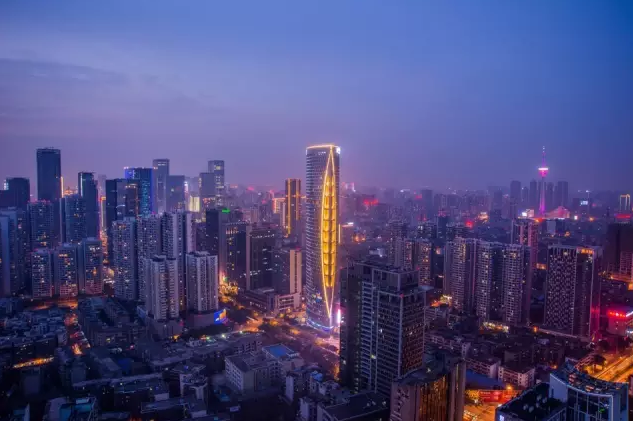我们看到了成都跨时代的改变，看到了成都满满的发展与进步，现在的成都 早已被速度与激情所填充，而成都人的追求也因此变得越来越高：讲究速度，追求激情，崇尚品质！在每一个大时代来临之际，我们总能感受到成都的活力与魄力，在每一次与国际事件的接轨中，我们都能看到成都这座城市在这个时代下所表现出的敢于尝试与拼搏的精神，而这种精神，恰好与美国火爆的NASCAR赛车盛事想要表现的敢于打破常规的态度如出一辙。因此， 他们选择了这里！讲究舒适，追求安逸，崇尚乐趣，是成都人生活的态度；讲究速度，追求激情，崇尚品质，是成都人做事的魄力。NASCAR是一场感受引擎轰鸣与极速的比赛，是一场拥有磅礴力量让人热血沸腾的比赛，是一场铿锵碰撞令人肾上腺素飚升的比赛。这场比赛，将再度证明，成都在这个时代下所具备着充足的速度与激情，让我们打破常规，共同感受NASCAR带来的激情！8月5日，直通美国NASCAR，雪佛兰·科鲁兹环行王者挑战赛（精英赛）将在成都正式打响，作为成都老司机的你，赶快做好准备，参与进这场狂野刺激的汽车赛事中来，当一回赛车英雄吧！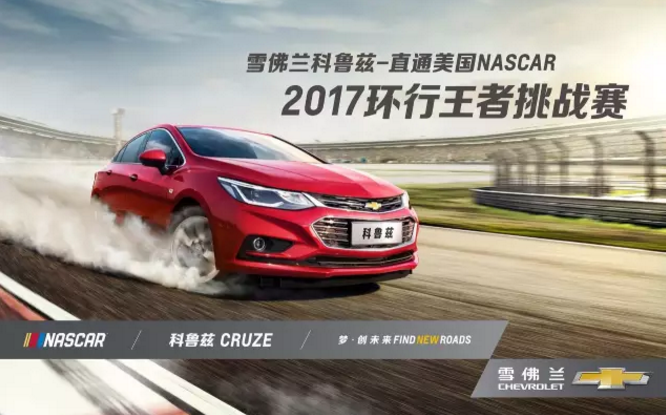NASCAR是轰动整个北美的赛车狂欢，能让人燃烧的赛车运动！ 它在美国火了70多年，每年都有超过1.2亿人次现场观赛。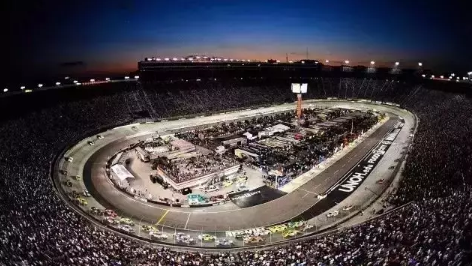300km/h的极速超车 3小时的高速追逐，赛车间疯狂肉搏 V8发动机凶猛咆哮。这些，你都能在全美最受欢迎，规模最大的赛车比赛“NASCAR”中一一目睹！而NASCAR中当之无愧的霸主，非雪佛兰莫属。在这场比赛当中，你除了能炫出毕生最强车技外，奖品也同样丰厚得让你根本停不下来！挑战赛积分总冠军直接获得第六代科迈罗RS为期一年使用权；亚军、季军获得科鲁兹为期一年使用权；除了冠亚季军外，2017年赛季积分前十还将送到美国现场，直接在美国看2019NASCAR赛车比赛！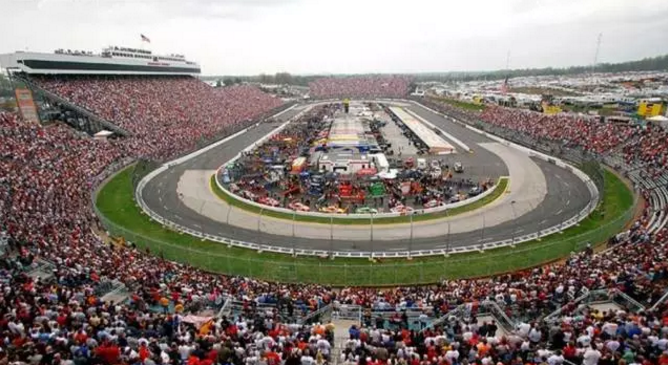作为此次挑战赛的唯一指定参赛车型，科鲁兹继承了雪佛兰品牌的赛车基因：一触即发的高效动力系统与结合纽博格林赛道调校级别的底盘造就了领先同级的卓越驾控品质。随着“环行王者挑战赛”的开启，科鲁兹凭借动力、操控、轴距等多方面的强劲实力，将为你带来更加自信和纯粹的驾控乐趣。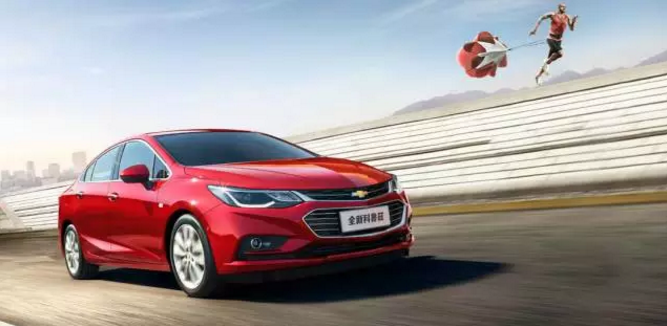传承“3C”家族设计风格，赛场上激情澎湃的瞬间，带来全面超越同级的驾控场面。六点式车身连接，加上欧宝专利技术的瓦特连杆设计，让科鲁兹拥有绝对的底盘操控能力。即使在如同NASCAR比赛的环形赛道上，科鲁兹也有更灵活的操控性，拥有超越同级车辆的稳定性能。同级领先的APA第二代智能泊车系统，配合同级唯一的4G LTE 移动Wifi，不是赛车手的老司机也能酷炫地装逼！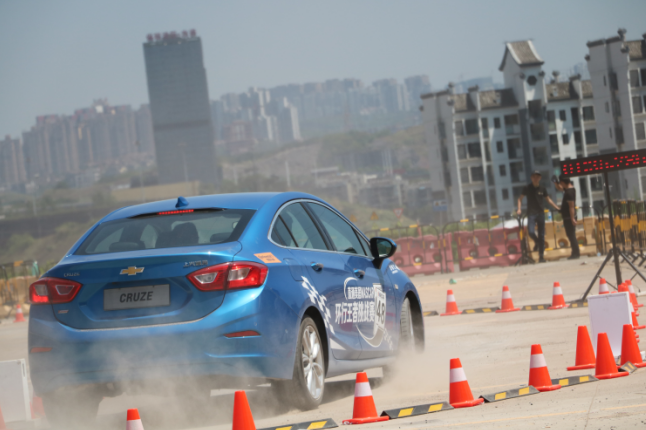全新科鲁兹搭载了 1.4T SIDI顶置直喷涡轮增压发动机，可以实现在低速段获得强劲动力并实现油门快速响应，约1500rpm时就可以获得约90%最大扭矩的输出，240N·m的最大扭矩会在2000-4000转持续输出。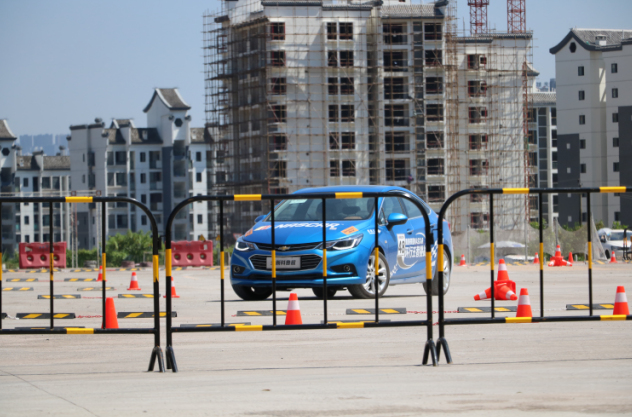此外，7速DCG双离合变速箱的变速比可达到7.47（一般6速变速箱速比仅为6.0），具备7个档位的紧凑3轴设计，更出色的动力传递性能，让换挡更平顺，更精准。提升低速动力响应与高速燃油经济性。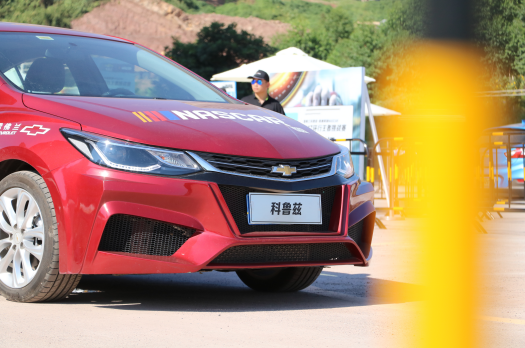只有在全面了解科鲁兹动力的基础上，才能在赛道上控制自如，做到真正的快准狠。赛车真正的意义在于追逐梦想，每个人都应该上一次赛道，释放心中狂野，感受狂飙极速，享受疾驰快感。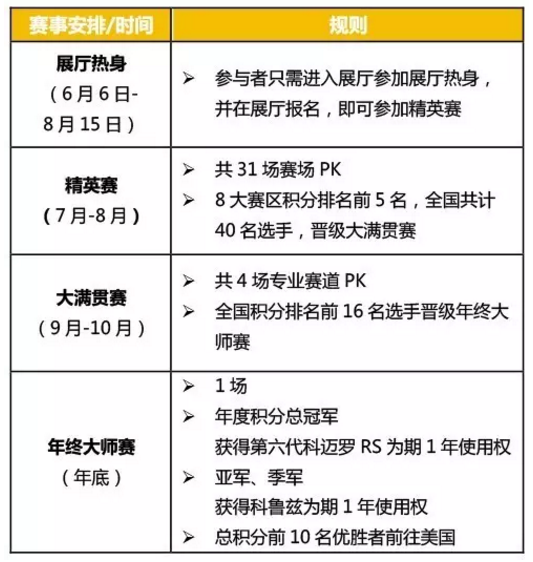<精英赛时间>

① 7月22日 重庆站  ② 8月5日  成都站

③ 8月5日  贵阳站   ④ 8月12日  昆明站

<大赛积分机制>

PS:积分除了能助老司机们一路晋级外，还可兑换丰厚礼品哦！通往美国NASCAR大赛的捷径已经开启，是时候展示你的高超技术了。参加活动有机会赢取迪士尼门票，更享多重购车礼遇！2017雪佛兰科鲁兹-直通美国NASCAR“环行王者挑战赛”8月5日在成都火力打响，你只需前往全国雪佛兰展厅或扫描下方二维码，即可预约报名。成都精英赛时间：8月5日；活动地点：成都幸福梅林体验中心。扫描二维码立即报名雪佛兰直通美国NASCAR西南精英赛。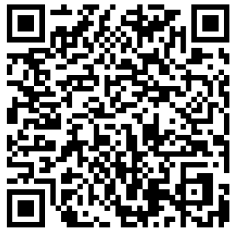##### 相关资讯

|分享

•已阅！握爪
•我手滑为你点赞
•128个赞！
•不明觉厉
•阅后既醉
•有钱！任性
•照片太美，我不敢看
•干货！杠杠的
•高大上
•膜拜中！
•请接受我的膝盖
•猫哥/猫妹么么哒
•神吐槽
•我只笑笑不说话
•我想静静
•窒息
##### 资讯相关车系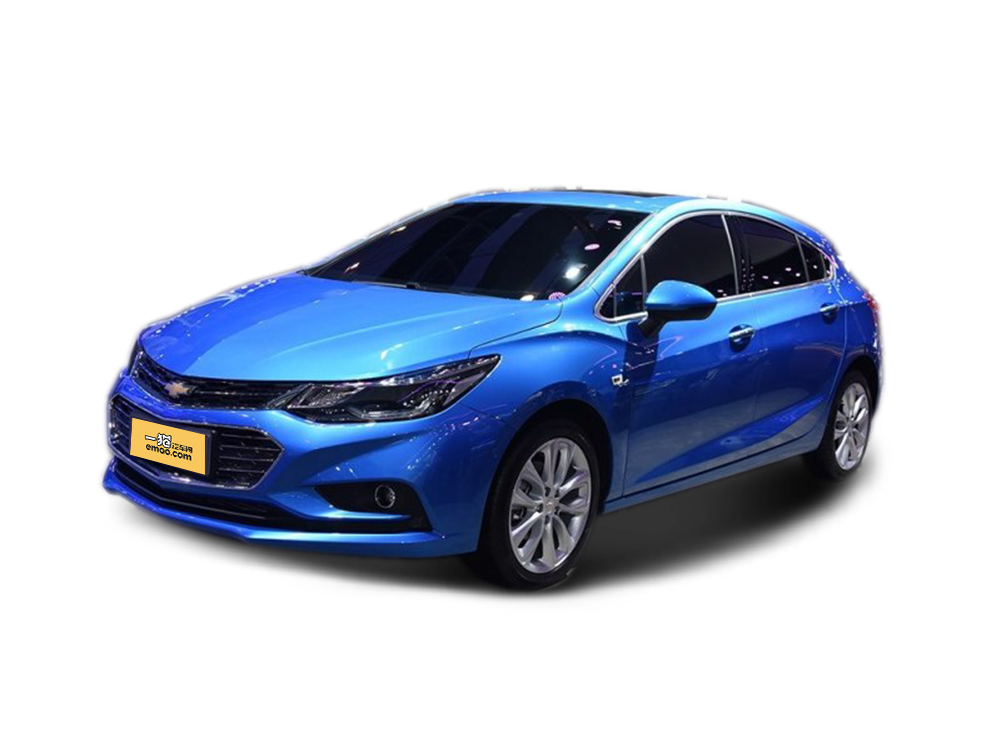##### 热度排行• 阿斯顿·马丁
• 奥迪
• 阿尔法罗密欧
• ALPINA

• 巴博斯
• 宝骏
• 宝马
• 保时捷
• 北汽制造
• 奔驰
• 奔腾
• 本田
• 比亚迪
• 标致
• 别克
• 宾利
• 布加迪
• 北汽威旺
• 北京
• 北汽绅宝
• 北汽幻速
• 北汽新能源
• 宝沃
• 比速汽车
• 北汽道达

• 昌河
• 长安
• 长城
• 长安商用
• 成功汽车
• 长江EV
• 长安轻车型

• 大众
• 道奇
• 东风
• 东风风神
• 东风小康
• 东南
• DS
• 东风风行
• 东风风度
• 东风风光

• 法拉利
• 菲亚特
• 丰田
• 福迪
• 福特
• 福田汽车
• 福汽启腾
• 风诺

• GMC
• 广汽传祺
• 广汽吉奥
• 观致

• 哈飞
• 海格
• 海马
• 华泰
• 黄海
• 恒天
• 红旗
• 哈弗
• 华颂
• 华凯
• 华泰新能源
• 汉腾汽车

• Jeep
• 江淮
• 江铃
• 捷豹
• 金杯
• 九龙
• 吉利汽车
• 金旅
• 金龙
• 江铃集团轻汽
• 江铃集团新能源
• 君马汽车
• 捷途

• 开瑞
• 凯迪拉克
• 科尼赛克
• 克莱斯勒
• KTM
• 卡威
• 凯翼
• 康迪
• 康迪电动汽车集团

• 猎豹汽车
• 兰博基尼
• 劳斯莱斯
• 雷克萨斯
• 雷诺
• 理念
• 力帆
• 莲花汽车
• 林肯
• 铃木
• 陆风
• 路虎
• 路特斯
• 领志
• 领克
• 零跑汽车

• MG
• MINI
• 马自达
• 玛莎拉蒂
• 迈凯伦
• 摩根

• 纳智捷

• 讴歌
• 欧宝
• 欧朗
• 欧拉
• 欧尚汽车

• 帕加尼

• 奇瑞
• 启辰
• 起亚
• 前途
• 庆铃汽车

• 日产
• 荣威
• 瑞麒汽车
• 如虎
• 瑞驰

• 上汽大通
• smart
• 三菱
• 双环
• 双龙
• 斯巴鲁
• 斯柯达
• 萨博
• 思铭
• 赛麟
• SWM斯威汽车

• TESLA
• 泰卡特
• 腾势

• 威麟
• 威兹曼
• 沃尔沃
• 五菱汽车
• 五十铃
• 潍柴英致
• WEY
• 蔚来
• 威马汽车

• 现代
• 雪佛兰
• 雪铁龙
• 西雅特
• 新特汽车
• 小鹏汽车
• 新宝骏

• 野马汽车
• 一汽
• 依维柯
• 英菲尼迪
• 永源
• 驭胜

• 中华
• 中兴
• 众泰
• 知豆
• 之诺
• 正道汽车
• A
• B
• C
• D
• E
• F
• G
• H
• I
• J
• K
• L
• M
• N
• O
• P
• Q
• R
• S
• T
• U
• V
• W
• X
• Y
• Z

• 阿斯顿·马丁
• 奥迪
• 阿尔法罗密欧
• ALPINA

• 巴博斯
• 宝骏
• 宝马
• 保时捷
• 北汽制造
• 奔驰
• 奔腾
• 本田
• 比亚迪
• 标致
• 别克
• 宾利
• 布加迪
• 北汽威旺
• 北京
• 北汽绅宝
• 北汽幻速
• 北汽新能源
• 宝沃
• 比速汽车
• 北汽道达

• 昌河
• 长安
• 长城
• 长安商用
• 成功汽车
• 长江EV
• 长安轻车型

• 大众
• 道奇
• 东风
• 东风风神
• 东风小康
• 东南
• DS
• 东风风行
• 东风风度
• 东风风光

• 法拉利
• 菲亚特
• 丰田
• 福迪
• 福特
• 福田汽车
• 福汽启腾
• 风诺

• GMC
• 广汽传祺
• 广汽吉奥
• 观致

• 哈飞
• 海格
• 海马
• 华泰
• 黄海
• 恒天
• 红旗
• 哈弗
• 华颂
• 华凯
• 华泰新能源
• 汉腾汽车

• Jeep
• 江淮
• 江铃
• 捷豹
• 金杯
• 九龙
• 吉利汽车
• 金旅
• 金龙
• 江铃集团轻汽
• 江铃集团新能源
• 君马汽车
• 捷途

• 开瑞
• 凯迪拉克
• 科尼赛克
• 克莱斯勒
• KTM
• 卡威
• 凯翼
• 康迪
• 康迪电动汽车集团

• 猎豹汽车
• 兰博基尼
• 劳斯莱斯
• 雷克萨斯
• 雷诺
• 理念
• 力帆
• 莲花汽车
• 林肯
• 铃木
• 陆风
• 路虎
• 路特斯
• 领志
• 领克
• 零跑汽车

• MG
• MINI
• 马自达
• 玛莎拉蒂
• 迈凯伦
• 摩根

• 纳智捷

• 讴歌
• 欧宝
• 欧朗
• 欧拉
• 欧尚汽车

• 帕加尼

• 奇瑞
• 启辰
• 起亚
• 前途
• 庆铃汽车

• 日产
• 荣威
• 瑞麒汽车
• 如虎
• 瑞驰

• 上汽大通
• smart
• 三菱
• 双环
• 双龙
• 斯巴鲁
• 斯柯达
• 萨博
• 思铭
• 赛麟
• SWM斯威汽车

• TESLA
• 泰卡特
• 腾势

• 威麟
• 威兹曼
• 沃尔沃
• 五菱汽车
• 五十铃
• 潍柴英致
• WEY
• 蔚来
• 威马汽车

• 现代
• 雪佛兰
• 雪铁龙
• 西雅特
• 新特汽车
• 小鹏汽车
• 新宝骏

• 野马汽车
• 一汽
• 依维柯
• 英菲尼迪
• 永源
• 驭胜

• 中华
• 中兴
• 众泰
• 知豆
• 之诺
• 正道汽车
• A
• B
• C
• D
• E
• F
• G
• H
• I
• J
• K
• L
• M
• N
• O
• P
• Q
• R
• S
• T
• U
• V
• W
• X
• Y
• Z

• 阿斯顿·马丁
• 奥迪
• 阿尔法罗密欧
• ALPINA

• 巴博斯
• 宝骏
• 宝马
• 保时捷
• 北汽制造
• 奔驰
• 奔腾
• 本田
• 比亚迪
• 标致
• 别克
• 宾利
• 布加迪
• 北汽威旺
• 北京
• 北汽绅宝
• 北汽幻速
• 北汽新能源
• 宝沃
• 比速汽车
• 北汽道达

• 昌河
• 长安
• 长城
• 长安商用
• 成功汽车
• 长江EV
• 长安轻车型

• 大众
• 道奇
• 东风
• 东风风神
• 东风小康
• 东南
• DS
• 东风风行
• 东风风度
• 东风风光

• 法拉利
• 菲亚特
• 丰田
• 福迪
• 福特
• 福田汽车
• 福汽启腾
• 风诺

• GMC
• 广汽传祺
• 广汽吉奥
• 观致

• 哈飞
• 海格
• 海马
• 华泰
• 黄海
• 恒天
• 红旗
• 哈弗
• 华颂
• 华凯
• 华泰新能源
• 汉腾汽车

• Jeep
• 江淮
• 江铃
• 捷豹
• 金杯
• 九龙
• 吉利汽车
• 金旅
• 金龙
• 江铃集团轻汽
• 江铃集团新能源
• 君马汽车
• 捷途

• 开瑞
• 凯迪拉克
• 科尼赛克
• 克莱斯勒
• KTM
• 卡威
• 凯翼
• 康迪
• 康迪电动汽车集团

• 猎豹汽车
• 兰博基尼
• 劳斯莱斯
• 雷克萨斯
• 雷诺
• 理念
• 力帆
• 莲花汽车
• 林肯
• 铃木
• 陆风
• 路虎
• 路特斯
• 领志
• 领克
• 零跑汽车

• MG
• MINI
• 马自达
• 玛莎拉蒂
• 迈凯伦
• 摩根

• 纳智捷

• 讴歌
• 欧宝
• 欧朗
• 欧拉
• 欧尚汽车

• 帕加尼

• 奇瑞
• 启辰
• 起亚
• 前途
• 庆铃汽车

• 日产
• 荣威
• 瑞麒汽车
• 如虎
• 瑞驰

• 上汽大通
• smart
• 三菱
• 双环
• 双龙
• 斯巴鲁
• 斯柯达
• 萨博
• 思铭
• 赛麟
• SWM斯威汽车

• TESLA
• 泰卡特
• 腾势

• 威麟
• 威兹曼
• 沃尔沃
• 五菱汽车
• 五十铃
• 潍柴英致
• WEY
• 蔚来
• 威马汽车

• 现代
• 雪佛兰
• 雪铁龙
• 西雅特
• 新特汽车
• 小鹏汽车
• 新宝骏

• 野马汽车
• 一汽
• 依维柯
• 英菲尼迪
• 永源
• 驭胜

• 中华
• 中兴
• 众泰
• 知豆
• 之诺
• 正道汽车
• A
• B
• C
• D
• E
• F
• G
• H
• I
• J
• K
• L
• M
• N
• O
• P
• Q
• R
• S
• T
• U
• V
• W
• X
• Y
• Z